## Statistical Views with Histograms and Box Plots in Python Pandas - statistical tools that will help you understand your data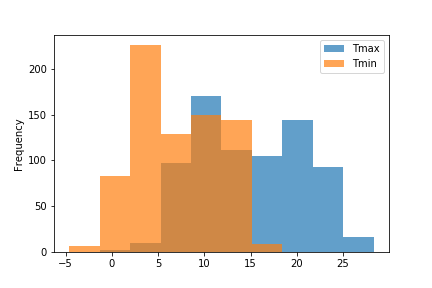We are going to use Jupyter Notebooks and Python with Pandas to give us a statistical view of our data.

You really don’t need to know much about programming but you will need to be able to understand just a little bit of Python.

Before you start you need to reasonably comfortable using Jupyter Notebooks. If you aren’t or don’t have Jupyter installed on your computer, don’t worry, I’ve written a tutorial that will show you how to install Jupyter and get you going. You can find it here: Setting Up Jupyter Notebooks for Data Visualization .

Also, you might want to take a look at a previous article about data visualization with Pandas that explores the use of line graphs, bar graphs, scatter diagrams and pie charts. It’s here: Simple Data Visualization with Pandas.

In this article we are going to explore the more data visualization capabilities of Pandas that are concerned with a statistical view of our data.

We’ll use Jupyter Notebooks to create the charts from a data set in the form of a CSV file.
We use Jupyter Notebooks because they allow us to experiment with the charts that we produce before exporting them for use in a document. They also allow us to create complete documents including those charts.

I often use a Jupyter Notebook for the draft versions of articles, including this one.

## Preparing for your statistical plots

First, you need to start up a Jupyter notebook (refer to the introductory article above, if you are unsure).

In your first code cell type in the following:

```import numpy as np
import pandas as pd
import matplotlib.pyplot as plt```

This will be familiar, if you have followed my previous article, it imports all of the necessary Python libraries to do data visualization with Pandas: numpy is a maths package, pandas gives us ways of manipulating data and matplotlib provides the basic plotting functionality that Pandas uses to produce charts and graphs.

Run this code once in order that all the subsequent bits of code will work.

## Getting the data for visualization

Before we start to visualize the data we need to load it from somewhere. I’ve provided a csv file of London weather data. There are two files, one is limited to 2018 (this was used in the previous article) and the other ranges from 1955 to early 2019. It’s a subset of historical data available from the Met Office in the UK and records the maximum and minimum temperatures, the rainfall and the number of hours of sun for each month.

The snippet of code below uses a variable weather to hold the weather data and we load the csv file into that variable from the url, as shown.

The second line of code is simply the variable name, and this displays the data as a table.
In the table you can see that the columns are labelled Year, Month, Tmax (Maximum temperature), Tmin (Minimum temperature), Rain (in millimetres) and Sun (hours of sunlight). This is the complete data set from 1955. (The subset from 2018 is called london2018.csv.)

```weather = pd.read_csv('https://coded2.herokuapp.com/datavizpandas/londonweather.csv')
weather```

The data table looks something like this but is rather bigger.

NOTE: When you are working with your own data, you can store the data file in the same directory as your notebook — then the code to load the data would look something like:

## Histograms - the first statistical view

The weather variable is a Pandas dataframe. This is essentially a table, as we saw above, but Pandas provides us with all sorts of functionality associated with the dataframe. One of these functions is the ability to plot a graph. We simply use the code weather.plot.hist() to create a histogram. We need to specify the values that we are interested in and we do this by referencing the column name from the dataframe thus:

`weather.plot.hist(y='Rain');`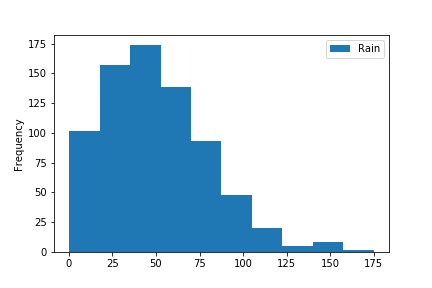Your first statistical visualization - a histogram

You can probably see that the data is grouped into 10 ranges, or bins. The first bar tells us that rainfall in the range 0 to an number approaching 25 occurred 100 times, and that the most frequent value was around 50.

If we want to see a little more detail, we can increase the number of bins. Here is the chart with 30 bins:

`weather.plot.hist(y='Rain', bins=30);`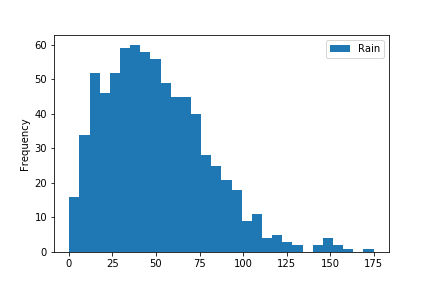A histogram with more bins

The shape is similar but because the range held in each bin is smaller, there are more steps and the frequency of each bin is lower.

As we increase the number of bins, we get more detail but tend to lose the overall picture. Here is the histogram with 100 bins.

`weather.plot.hist(y='Rain', bins=100);`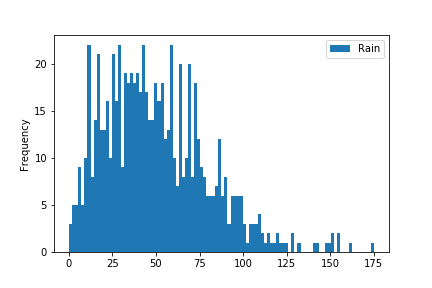More bins

We can draw a horizontal histogram by specifying its orientation as ‘horizontal’.

`weather.plot.hist(y='Rain', bins=10, orientation='horizontal');`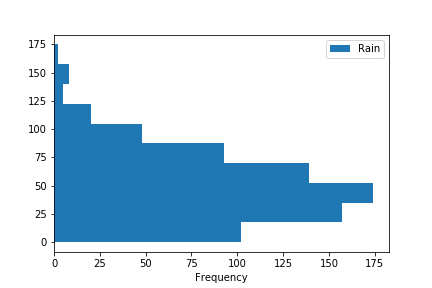A horizontal histogram

We can plot more than one histogram in the same graph if we wish, by listing the columns that we are interested in. Here is one that plots both the maximum and minimum temperatures.

You can see that maximum temperatures above 25 degrees are not that common and neither are minimums below zero.

`weather.plot.hist(y=['Tmax','Tmin'], bins=10)`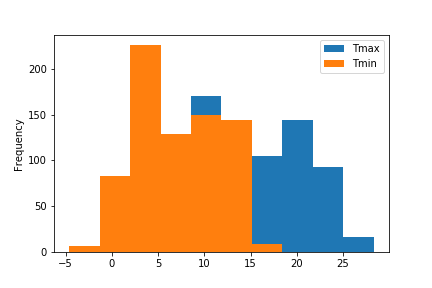Multiplot histogram

In this graph, one histogram obscures the other, so we cannot see the left side of Tmax. We can cure this by adjusting the transparency of the histograms. We do this by specifying an alpha value. This can take a value between zero and one, where 1 is opaque and 0 is completely transparent. I’ve chosen a value of 0.7 and you can see the result below — Tmax is now visible ‘behind’ Tmin.

`weather.plot.hist(y=['Tmax','Tmin'], bins=10, alpha=0.7)`Multiplot histogram

Or we could plot the histograms as separate graphs by specifying subplots to be True and also defining the layout and size a shown below. You can play around with the value of layout and figsize to get the size and shape that you are happy with.

`weather.plot.hist(y=['Tmax','Tmin'],subplots=True, layout=(1,2), figsize=(10,5));`

## Boxplots - a second statistical view

A boxplot gives specific statistical information about our data. Consider the plot below for Tmax.

`weather.plot.box(y=['Tmax']);`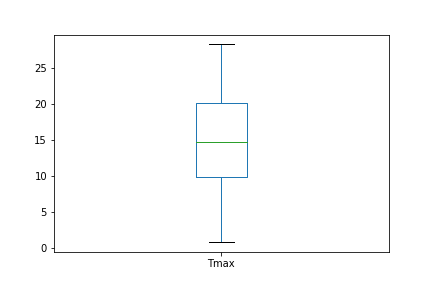Boxplot

This simple diagram holds a lot of information. The box, itself, represents the data points that fall between the 25th and 75th percentiles, the line across the box represents the mean of the data, while the ‘whiskers’ that extend above and below the box give us the maximum and minimum values.

We can confirm that the box does indeed gives us this information by using the describe function for a column of a dataframe.

`weather['Tmax'].describe()`

When we run this code we get this as an output:

```count    748.000000
mean      14.953610
std        5.783022
min        0.800000
25%        9.800000
50%       14.700000
75%       20.100000
max       28.300000
Name: Tmax, dtype: float64```

This function tells about the column that we have specified and confirms the values that we can see in the box plot, for example the mean is approximately 15 and the maximum and minimum values are approximately 1 and 28, respectively.

To compare two columns, we can use a subplot, similar to what we saw, above.

Here we have Tmin and Tmax compared and, while the scale is different, we can see that the range of values for each column is not too different, although Tmax varies a little more.

`weather.plot.box(y=['Tmax','Tmin'],subplots=True,layout=(1,2), figsize=(10,5));`

## Scatter Plot

We looked at the scatter plot in the previous article, (it plots a series of points that correspond to two variables and allows us to determine if there is a relationship between them) but with the limited data we had it was difficult to spot a relationship. Now we have more data it’s worth re-visiting them.
The scatter plot below plots Sun and Tmax and you can clearly see the relationship between the two. As the number of hours of sun increases, so does the maximum temperature. Which is, of course, what we would expect — generally speaking the more sun we get the hotter it is.

`weather.plot.scatter(x='Sun', y='Tmax');`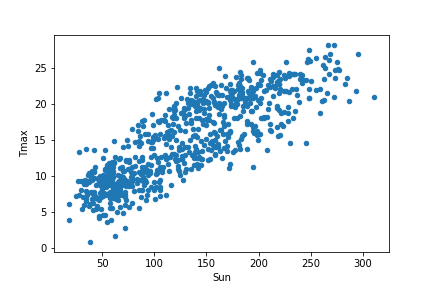Scatter plot

## Saving the Charts

This was also covered in the previous article but bears repeating as it is useful (and quite short).
You normally want to be able to use the charts that you produce. If you want to use them in a presentation or document, then it would be useful to be able to export them as image files that you can include in another file.

The simple way of saving the images is like this:

```weather.plot.scatter(x='Sun', y='Tmax');
plt.savefig("suntmaxscatter.png")```

The variable plt is created when you plot a graph and it has a function called savefig which is used to save the image. You can see that the name of the file is specified inside the brackets and in this case it will save to a file called “suntmaxscatter.png” in the same directory as the notebook.

This was an introduction to histograms and boxplots with Pandas and Jupyter Notebooks. We’ve seen how we can produce a range of charts from a data file and save them for use in our documents.